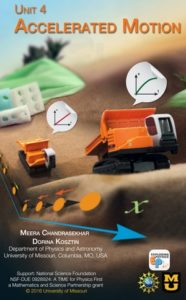### eUnit 4 Accelerated MotionBuilding on knowledge from previous units, students connect uniform motion concepts to accelerated motion. Students design their own experiments using toy cars, ramps, and spark timers. In these experiments students collect data, build position-time graphs, interpret slope, and learn the concepts of instantaneous velocity and acceleration. They make motion diagrams for objects that speed up or slow down and compare accelerated motion with uniform motion. To measure and analyze position, velocity and acceleration of an object moving on an incline, students use technology (timers, photogates and motion detectors) to collect data. By the end of the unit, students will be able to answer the following question:

• What does the position-time graph of a car traveling down an incline look like?
• How does one get a velocity-time graph from a position-time graph for motion with increasing speed?
• What does the slope of the velocity-time graph represent?
• How can we experimentally obtain a velocity-time graph?
• How do you pictorially represent accelerated motion?
• How do you mathematically calculate the factors involved in accelerated motion?
• How can you predict where two cars meet?

Suggested timeline for teaching Unit 4

Big Ideas
1. Uniform accelerated motion means that velocity changes by equal amounts in equal time intervals.
2. An object that accelerates is speeding up, slowing down, or turning.
3. Motion can be described in different ways: with words, graphs, motion diagrams and mathematical models.

Learning Goals and Objectives
By the end of this unit, the students should be able to:

1. From a conducted experiment on an object in uniform accelerated motion, analyze the change in position, time, velocity and acceleration of the object. (DOK4) a) Compare and contrast the x vs. t graphs for uniform accelerated motion with those for uniform motion. b) Compare and contrast the slopes of x vs. t graphs for uniform accelerated motion with those for uniform motion. c) Distinguish between instantaneous and average velocity. d) Determine the instantaneous velocity of the object at a given time. e) Compare and contrast the v vs. t graphs for uniform accelerated motion with those for uniform motion. f) Compare and contrast the slopes of v vs. t graphs for uniform accelerated motion with those for uniform motion. g) Determine the acceleration of the object from the slope of the v vs. t graph. h) Use technology (i.e., motion detectors) to measure accelerated motion and produce motion graphs. i) Compare motion for objects undergoing different accelerations.
2. Describe an object in uniform accelerated motion using multiple representations (i.e., verbal descriptions, motion diagrams, graphs and mathematical models). (DOK3) a) Verbally describe the motion of an object in uniform accelerated motion. b) Draw a motion diagram for an object in uniform accelerated motion. c) Construct x vs. t, v vs. t and a vs. t graphs and interpret data. d) Mathematically describe acceleration as the slope of a v vs. t graph (a = Dv/Dt). e) Convert among verbal descriptions, motion diagrams, graphs and mathematical models.
3. Mathematically and graphically determine the displacement, velocity and acceleration of an object in uniform accelerated motion. (DOK3) a) Construct the corresponding v vs. t graph from a given x vs. t graph. b) Construct the corresponding x vs. t graph from a given v vs. t graph. c) Construct the corresponding a vs. t graph from a given v vs. t graph. d) Construct the corresponding v vs. t graph from a given a vs. t graph. e) Determine the instantaneous velocity of an accelerating object two ways: i) Determine the slope of the secant or tangent to an x vs. t graph at a given point. ii) Use the mathematical expression vf = vi + aΔt. f) Determine the displacement of an accelerating object two ways: i) Find the area under a v vs. t graph. ii) Using the mathematical expression Δx = viΔt + a(Δt)2/2. g) Determine the acceleration of an accelerating object four ways: i) Find the slope of a v vs. t graph. ii) Use the mathematical expression, iii) Rearrange the mathematical expression, vf = vi + aΔt. iv) Rearrange the mathematical expression, Δx = viΔt + a(Δt)2/2
4. Design and conduct experiments to study uniform accelerated motion. (DOK4) a) Make observations. b) Operationally define the variables. c) Generate a hypothesis. d) List the steps of the procedure used to collect the data. e) Collect the data and organize it into a table. f) Convert metric units as needed. g) Graph and interpret the results. h) Develop conclusions based on results.